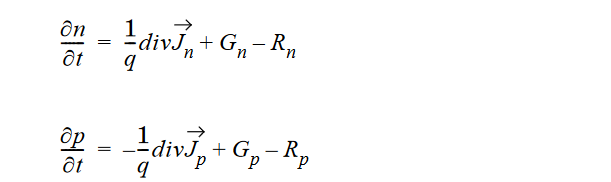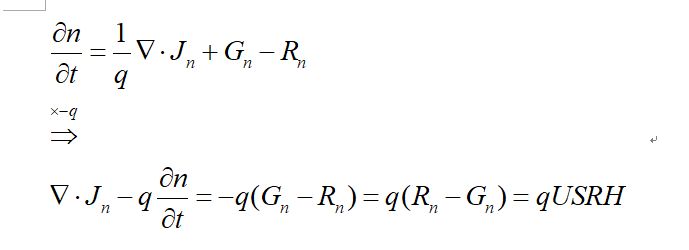Hello, Juan!
Sorry to bother you. But I am still confused about the positive/negative sign in the SRH model equation assembly.
We aimed to create the continuity equation about the holes and electron:This part code in Devsim is：

def CreateSRH(device, region):
USRH="(Electrons*Holes - n_i^2)/(taup*(Electrons + n1) + taun*(Holes + p1))"
Gn = "-ElectronCharge * USRH"
Gp = "+ElectronCharge * USRH"
CreateNodeModel(device, region, "USRH", USRH)
CreateNodeModel(device, region, "ElectronGeneration", Gn)
CreateNodeModel(device, region, "HoleGeneration", Gp)
for i in ("Electrons", "Holes"):
CreateNodeModelDerivative(device, region, "USRH", USRH, i)
CreateNodeModelDerivative(device, region, "ElectronGeneration", Gn, i)
CreateNodeModelDerivative(device, region, "HoleGeneration", Gp, i)

def CreateECE(device, region,mu_n):
CreateElectronCurrent(device, region,mu_n)
NCharge = "-ElectronCharge * Electrons"
CreateNodeModel(device, region, "NCharge", NCharge)
CreateNodeModelDerivative(device, region, "NCharge", NCharge, "Electrons")

equation(device=device, region=region, name="ElectronContinuityEquation", variable_name="Electrons",
time_node_model = "NCharge",
edge_model="ElectronCurrent", variable_update="positive", node_model="ElectronGeneration")


So the USRH=Rn-Gn or USRH=Gn-Rn. In the book “Analysis and Simulation of Semiconductor Devices - S. Selberherr”, this expression should represent Rn-Gn:

So Positive USRH means recombination and negative R means generation. We can get the equation using USRH:However, the code define Gn=-q*USRH. And the ECE equation in:

    equation(device=device, region=region, name="ElectronContinuityEquation", variable_name="Electrons",
time_node_model = "NCharge",
edge_model="ElectronCurrent", variable_update="positive", node_model="ElectronGeneration")


It seems create an equation:

So I’m getting a contradictory result here, and I don’t know where the problem is. I hope you can explain this part of the code to me, and what exactly is the form of the equation here assembled.

Hi @ghost

In DEVSIM the equations are solved so that the right hand side is 0 and all of the terms in the equation are on the LHS:

We adopted the convention that \bf{J_n} is in the direction oppositie of electron flow.

Also:

\text{NCharge} = -q \cdot n \\ \text{PCharge} = q \cdot p \\

So that the formulation we provided is:

-q \frac{\partial{n}}{\partial{t}} + \nabla \cdot {\bf{J}_n} + q G_n = 0 \\ +q \frac{\partial{p}}{\partial{t}} + \nabla \cdot {\bf{J}_p} + q G_p = 0

where the generation terms have the same signs as the time derivative terms for the accumulation of electrons and holes, respectively.

or

-q \frac{\partial{n}}{\partial{t}} + \nabla \cdot {\bf{J}_n} - q \cdot U_{SRH} = 0 \\ +q \frac{\partial{p}}{\partial{t}} + \nabla \cdot {\bf{J}_p} + q \cdot U_{SRH} = 0

Thanks, Juan. I have no problem now.

I apologize for all of the edits. Correcting the signs is tricky

Please let me know if there is a mistake in any of the signs in the implementation or this description.